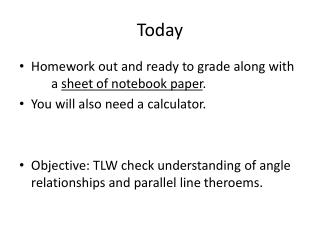DownloadDownload PresentationToday

# Today

Télécharger la présentation## Today

- - - - - - - - - - - - - - - - - - - - - - - - - - - E N D - - - - - - - - - - - - - - - - - - - - - - - - - - -
##### Presentation Transcript

1. Today • Homework out and ready to grade along with a sheet of notebook paper. • You will also need a calculator. • Objective: TLW check understanding of angle relationships and parallel line theroems.

2. Today • Discuss answers and reasoning • Number your paper #1-22 • Work quietly as there are other classes working • At most 2 people at a question at a time.

3. What is the relationship between angles 2 and 7?

4. What is the relationship between angles 5 and 7?

5. What is the relationship between angles 1 and 8?

6. What is the relationship between angles 6 and 7?

7. What is the relationship between angles 1 and 7?

8. Fill in the blank with parallel, perpendicular, or skew.Line j and line k are ____

9. Fill in the blank with parallel, perpendicular, or skew.Line jand line m are ____

10. Fill in the blank with parallel, perpendicular, or skew.Line kand line m are ____

11. Solve for x

12. Solve for x

13. Solve for x

14. Solve for the variable

15. Solve for the variable

16. Find the measure of <1 and <2

17. Find the measure of <1 and <2

18. Find the measure of <1 and <2

19. Solve for x

20. Can you say that line l and line k are parallel?

21. Can you say that line j and line k are parallel?

22. Can you say that line m and line n are parallel?

23. Can you say that line m and line n are perpendicular?